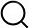# Also in the Article

Measurement of sulfur isotope enrichments
This protocol is extracted from research article:
Large sulfur isotope fractionation by bacterial sulfide oxidation

Procedure

The microbial cultivation samples were analyzed for sulfur isotopes following the method described in (40). Sulfur isotopic ratios were reported as(6)where 34R = 34S/32S and V-CDT refers to the Vienna-Canyon Diablo Troilite international reference scale. The uncertainty on δ34SSO4 was determined using the SD of the standard NBS 127 at the beginning and the end of each run (less than 0.3‰, 1σ). Measurements of δ34SSO4 and δ34SH2S were calibrated according to the following standards: NBS 127, IAEA-SO-6, IAEA-SO-5, IAEA-S-2, IAEA-S3, and an in-house silver sulfide standard with δ34SSO4 of 20.3, −34.1, 0.5, 22.3, −34.3, and 3.4‰, respectively. δ34S is reported with respect to V-CDT. The measurement of sulfur isotope fractionation factor during sulfide oxidation was estimated as the regression of δ34S versus −ln(f) for the reactant (41)$δR=δR0+εP/Rln(f)$(7)where δR is the measured isotopic composition of the reactant sulfide, δR0 is the initial isotopic composition of the reactant sulfide, f is the fraction of reactant consumed over the initial amount of reactant, and εP/R is the sulfur isotope fractionation factor between the reactant, R, and the product, P. The sulfur isotope fractionation was also estimated using the regression of the product on a plot of δ34S versus [f/(1 − f)]ln(f) (41)$δp=δR0−εP/R[f/(1−f)]ln(f)$(8)where δp is the measured isotopic composition of the product sulfate and also provides an estimate of the fractionation factor. Uncertainty on εP/R is reported as the SD on the linear regressions. In the experiments with cells from the stationary phase (A to D), specific fractionation factors were reported with regard to the maximum difference between the δ34S of sulfate and that of sulfide.

Note: The content above has been extracted from a research article, so it may not display correctly.

Q&A